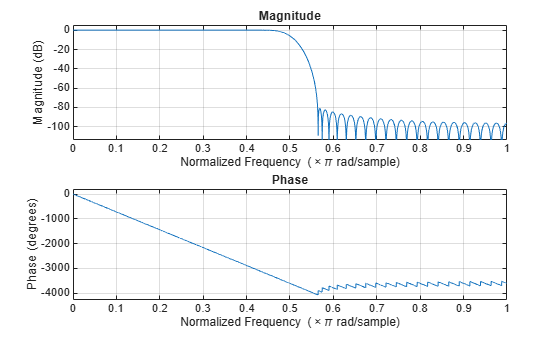Documentation

freqz

Frequency response of filter

Description

[h,w] = freqz(sysobj) returns the complex frequency response h of the filter System object™, sysobj. The vector w contains the frequencies (in radians/sample) at which the function evaluates the frequency response. The frequency response is evaluated at 8192 points equally spaced around the upper half of the unit circle.

[h,w] = freqz(sysobj,n) returns the complex frequency response of the filter System object and the corresponding frequencies at n points equally spaced around the upper half of the unit circle.

freqz uses the transfer function associated with the filter to calculate the frequency response of the filter with the current coefficient values.

[h,w] = freqz(sysobj,'Arithmetic',arithType) analyzes the filter System object, based on the arithmetic specified in arithType, using either of the previous syntaxes.

example

freqz(sysobj) uses fvtool to plot the magnitude and unwrapped phase of the frequency response of the filter System object sysobj.

For more input options, see freqz.

Examples

collapse all

This examples plot the frequency response of the lowpass FIR filter using freqz.

b = fir1(80,0.5,kaiser(81,8));
firFilt = dsp.FIRFilter('Numerator',b);
freqz(firFilt);Input Arguments

collapse all

Number of points over which the frequency response is computed. For an FIR filter where n is a power of two, the computation is done faster using FFTs.

Data Types: single | double | int8 | int16 | int32 | int64 | uint8 | uint16 | uint32 | uint64

Specify the arithmetic used during analysis. When the arithmetic input is not specified and the filter System object is unlocked, the analysis tool assumes a double-precision filter. The 'Arithmetic' property set to 'Fixed' applies only to filter System objects with fixed-point properties.

Output Arguments

collapse all

Complex n-element frequency response vector. If n is not specified, the function uses a default value of 8192. The frequency response is evaluated at n points equally spaced around the upper half of the unit circle.

Data Types: double
Complex Number Support: Yes

Frequency vector of length n, in radians/sample. w consists of n points equally spaced around the upper half of the unit circle (from 0 to π radians/sample). If n is not specified, the function uses a default value of 8192.

Data Types: double

Tips

There are several ways of analyzing the frequency response of filters. freqz accounts for quantization effects in the filter coefficients, but does not account for quantization effects in filtering arithmetic. To account for the quantization effects in filtering arithmetic, refer to function noisepsd.

Algorithms

freqz calculates the frequency response for a filter from the filter transfer function Hq(z). The complex-valued frequency response is calculated by evaluating Hq(ejω) at discrete values of w specified by the syntax you use. The integer input argument n determines the number of equally-spaced points around the upper half of the unit circle at which freqz evaluates the frequency response. The frequency ranges from 0 to π radians per sample when you do not supply a sampling frequency as an input argument. When you supply the scalar sampling frequency fs as an input argument to freqz, the frequency ranges from 0 to fs/2 Hz.